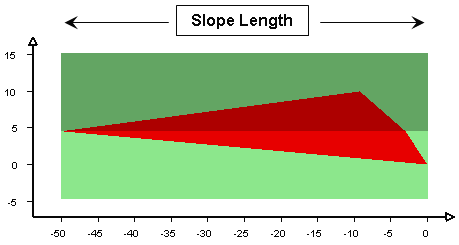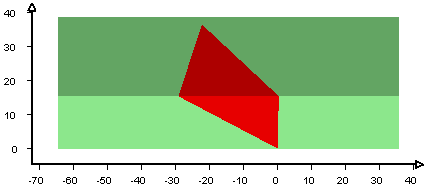The Rocscience International Conference 2021 Proceedings are now available. Read Now

# Slope Length

The Slope Length in SWedge is an optional input parameter, which can be used to limit or scale the wedge size. It can also be used as a display option.

• If the Slope Length is NOT specified, then SWedge assumes that the length dimension of the slope is sufficient to accommodate the maximum wedge size computed for the slope (i.e. by default, the Slope Length is unlimited).
• If you wish to limit the size of wedges based on the length dimension of the slope, then select the Slope Length check box in the Input Data dialog, and enter a value of slope length. This will ensure that the wedge size does not exceed the user-defined length of the slope. In general, you can use the Slope Length option to limit or scale the wedge size according to this dimension.

For example, in the model below, a Slope Length of 50 meters has been specified, and the wedge size has been scaled accordingly. The Slope Length limits the maximum extent of the wedge, as measured along the crest of the slope.

Definition of Slope Length used for wedge sizing in SWedgeNOTES:

• The Slope Length is always measured along the crest of the slope (i.e. along the line of intersection of the two slope planes). This is true even if the crest of the slope is not horizontal (i.e. the slope and upper face have different dip directions).
• Remember that the Slope Length is a limiting value. If the current wedge size is already smaller than the specified Slope Length, then the wedge size will not be affected.
• If the Slope Length is not specified, it is possible to generate wedges which have a very "long" dimension along the length of the slope (for example, if one of the joint planes has a strike which is nearly parallel to the strike of the slope planes). This can result in wedges which have unrealistic dimensions. In this case it may be necessary to use the Slope Length option to limit the wedge size.

## Slope Length in a Probabilistic or Combinations Analysis

A user-defined Slope Length is recommended in a Probabilistic Analysis, to ensure that all wedges generated by the statistical sampling of joint orientations will be limited in size by the Slope Length. If a Slope Length is not defined, then the Probabilistic Analysis may generate unrealistically long wedges, which may influence the analysis results.

For the same reasons, the Slope Length option is also recommended for a Combination Analysis.

## Slope Length as a Display Option

When you specify a Slope Length, the SWedgemodel is drawn using the specified Slope Length. Therefore, even if you are not using the Slope Length to scale the wedge size, you can use the Slope Length to display the actual slope length, or to adjust the appearance of the model for screen capture purposes. For example, in the model below, a Slope Length of 100 meters has been specified for display purposes; the wedge size is not affected.

User defined slope length = 100 metersIf the Slope Length is NOT specified, then the graphical (apparent) slope length is automatically calculated by SWedgebased on the current wedge size.